Ratio
Geometric Mean
Similarity
N-Solve
RANDOM
100

What are the two things compared in the following question:

Michael can run 5 miles in 60 minutes. How many miles can he run in 80 minutes?

Miles to Minutes

100

Draw a picture of the Geometric Mean on a Triangle.100

solve for x.x=12

100

EXPLAIN the steps of solve for nSolve on a CALCULATOR (not iPad)

2) press 3 (algebra), then press 1 (numerical solve).

3) Just plug in your equation.

100

Find WU.WU= 5.099

200

What are the THREE different symbols/ways to write a ratio?

Colon:

Division Line/

___ to ___

200

Find the geometric mean of the triangle.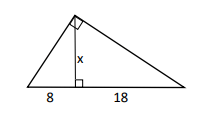12!

200

Solve for x.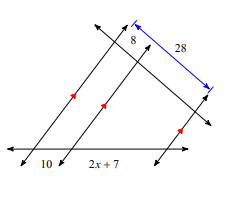x=9

200

Solve for x.x=78

200

Find a.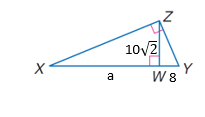a=25

300

A 5.6 foot man casts a shadow of 8.3 feet. How tall is a building if at that given moment it casts a shadow of 203.4 feet? Round to the nearest tenth.

The building is 137.2 feet tall!

300

Find the geometric mean between 36 and 49.

42

300

Are the following shapes similar to each other?NO. (34/15) does not equal (34/22)

300

Solve for x.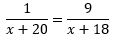x= -20.25

300

Find x.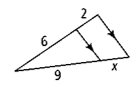x=3

400

The sides of a triangle, with a perimeter of 104 feet, have a ratio of 3:4:6. What is the length of each side?

24:32:48

400

(Round to 2 decimal places.)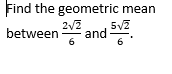0.75

400

Find x.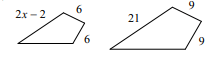x=8

400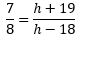h= -278

400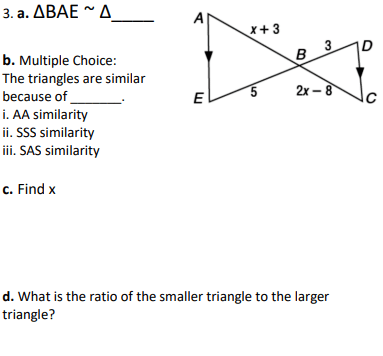a. BCD

b. AA Similarity

500

Two number have a ratio of 2 to 5. If their sum is 84 find the numbers.

24 and 60

500

sqrt12

is the geometric mean between x and y. Find x if

y = 3.

x=4

500

Solve for x.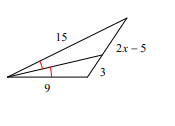x=5

500

Solve for b.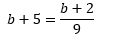b=-5.375

500

Find x and y.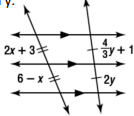x=1

y=1.5

Click to zoom